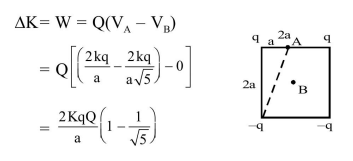Deepak Scored 45->99%ile with Bounce Back Crack Course. You can do it too!

# Two positive charges of magnitude 'q' are placed at the ends of a side (side 1 ) of a square of side '2a'.

Question:

Two positive charges of magnitude ' $q$ ' are placed at the ends of a side (side 1 ) of a square of side '2a'. Two negative charges of the same magnitude are kept at the other corners. Starting from rest, if a charge $\mathrm{Q}$ moves from the middle of side 1 to the centre of square, its kinetic energy at the centre of square is :-

1. $\frac{1}{4 \pi \epsilon_{0}} \frac{2 \mathrm{qQ}}{\mathrm{a}}\left(1-\frac{1}{\sqrt{5}}\right)$

2. Zero

3. $\frac{1}{4 \pi \in_{0}} \frac{2 \mathrm{qQ}}{\mathrm{a}}\left(1+\frac{1}{\sqrt{5}}\right)$

4. $\frac{1}{4 \pi \in_{0}} \frac{2 \mathrm{qQ}}{\mathrm{a}}\left(1-\frac{2}{\sqrt{5}}\right)$

Correct Option: 1

Solution: